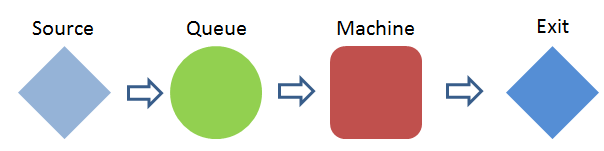# ManPy Single Server Example¶

In this example we will see how to create a model of ManPy objects.

Our model has the following structure:## Deterministic Model

The first implementation of the model is deterministic.

As values we have the following:
• The Source produces Parts. One part is produced every 30 seconds
• The capacity of Queue is 1
• The Machine processes one part at a time. The processing time is 15 seconds
• We want to study the system for 24 hours

First we have to import the appropriate ManPy components:

In :
from dream.simulation.imports import Source, Queue, Machine, Exit
from dream.simulation.Globals import runSimulation


Then, declare the instances of the model

In :
#define the objects of the model
S=Source('S1','Source',interarrivalTime={'distributionType':'Fixed','mean':0.5}, entity='Dream.Part')
Q=Queue('Q1','Queue', capacity=1)
M=Machine('M1','Machine', processingTime={'distributionType':'Fixed','mean':0.25})
E=Exit('E1','Exit')


Note that ManPy time units are decimals. So 30 and 15 seconds have been translated to 0.5 and 0.25 minutes respectively.

Next, we need to define the relationships of the objects, i.e. how they are connected in the model. We do this using the CoreObject.defineRouting method:

In :
#define predecessors and successors for the objects
S.defineRouting(successorList=[Q])
Q.defineRouting(predecessorList=[S],successorList=[M])
M.defineRouting(predecessorList=[Q],successorList=[E])
E.defineRouting(predecessorList=[M])


defineRouting in most CoreObjects gets two lists as arguments (perdecessorList, successorList) with this sequence. In special cases like the Source and the Exit only one list is required.

Now we are ready to run the simulation:

In :
# call the runSimulation giving the objects and the length of the experiment
runSimulation(objectList=[S,Q,M,E], maxSimTime=1440.0)


runSimulation is invoked with tow arguments. A list with all the ManPy simulation objects and the length of the experiment. There are other optional arguments as we will see in other examples.

After the simulation run, we may want to perform calculations on metrics:

In :
# calculate metrics
working_ratio = (M.totalWorkingTime/1440.0)*100


We used the Machine.totalWorkingTime attribute.

Finally, we output the results of the simulation run:

In :
#print the results
print "the system produced", E.numOfExits, "parts"
print "the total working ratio of the Machine is", working_ratio, "%"

the system produced 2880 parts
the total working ratio of the Machine is 50.0 %



Given the simplicity and the deterministic nature of the model, we can establish that the results are valid.

## Stochastic Model

In order to turn the result into stochastic, we will change the declaration of the objects.

Let's say that:
• The interarrival time of Parts in Source follows the Exponential distribution with an average of 30 seconds
• The processing time in Machine follows the Normal distribution with mean of 15 seconds and standard deviation of 30 seconds. We also truncate the distribution setting minimum=0 and maximum=3 minutes
In :
#define the objects of the model
S=Source('S1','Source',interarrivalTime={'distributionType':'Exp','mean':0.5}, entity='Dream.Part')
Q=Queue('Q1','Queue', capacity=1)
M=Machine('M1','Machine', processingTime={'distributionType':'Normal','mean':0.25,'stdev':0.5,'min':0,'max':3})
E=Exit('E1','Exit')


The routing will remain the same:

In :
#define predecessors and successors for the objects
S.defineRouting(successorList=[Q])
Q.defineRouting(predecessorList=[S],successorList=[M])
M.defineRouting(predecessorList=[Q],successorList=[E])
E.defineRouting(predecessorList=[M])


Now we can run the simulation. Note that we give the extra argument numberOfReplications=5 (default is 1 replication).

In :
# call the runSimulation giving the objects and the length of the experiment
runSimulation(objectList=[S,Q,M,E], maxSimTime=1440.0, numberOfReplications=5)


Now we print the E.Exits attribute that is a list of the number of exits pre replication.

In :
#print the results
print "Exits per experiment", E.Exits

Exits per experiment [2823, 2820, 2830, 2835, 2763]



This result can be used for statistical interference, e.g. calculation of Confidence Intervals.

## Full Examples

below is the full code of the examples, so that the user can easier manipulate them.

### Deterministic Model

In :
from dream.simulation.imports import Source, Queue, Machine, Exit
from dream.simulation.Globals import runSimulation

#define the objects of the model
S=Source('S1','Source',interarrivalTime={'distributionType':'Fixed','mean':0.5}, entity='Dream.Part')
Q=Queue('Q1','Queue', capacity=1)
M=Machine('M1','Machine', processingTime={'distributionType':'Fixed','mean':0.25})
E=Exit('E1','Exit')

#define predecessors and successors for the objects
S.defineRouting(successorList=[Q])
Q.defineRouting(predecessorList=[S],successorList=[M])
M.defineRouting(predecessorList=[Q],successorList=[E])
E.defineRouting(predecessorList=[M])

# call the runSimulation giving the objects and the length of the experiment
runSimulation(objectList=[S,Q,M,E], maxSimTime=1440.0)

# calculate metrics
working_ratio = (M.totalWorkingTime/1440.0)*100

#print the results
print "the system produced", E.numOfExits, "parts"
print "the total working ratio of the Machine is", working_ratio, "%"

the system produced 2880 parts
the total working ratio of the Machine is 50.0 %



### Stochastic Model

In :
from dream.simulation.imports import Source, Queue, Machine, Exit
from dream.simulation.Globals import runSimulation

#define the objects of the model
S=Source('S1','Source',interarrivalTime={'distributionType':'Exp','mean':0.5}, entity='Dream.Part')
Q=Queue('Q1','Queue', capacity=1)
M=Machine('M1','Machine', processingTime={'distributionType':'Normal','mean':0.25,'stdev':0.5,'min':0,'max':3})
E=Exit('E1','Exit')

#define predecessors and successors for the objects
S.defineRouting(successorList=[Q])
Q.defineRouting(predecessorList=[S],successorList=[M])
M.defineRouting(predecessorList=[Q],successorList=[E])
E.defineRouting(predecessorList=[M])

# call the runSimulation giving the objects and the length of the experiment
runSimulation(objectList=[S,Q,M,E], maxSimTime=1440.0, numberOfReplications=5)

#print the results
print "Exits per experiment", E.Exits

Exits per experiment [2849, 2819, 2828, 2780, 2763]


In []: